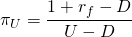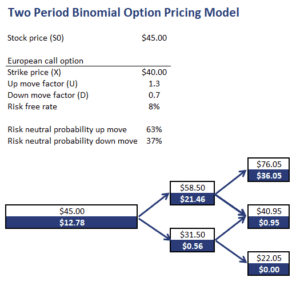# Two Period Binomial Option Pricing Model

The two period binomial option pricing model is a very popular model that explains how to price stock options. The model uses a so-called binomial model. A binomial model is based on the idea that, over the next period, the value of an asset can be equal to one of two possible values. Hence, the name binomial. The model can be used for pricing american style options.

On this page, we discuss the binomial model, discuss a two period binomial model example and finally implement a two period binomial option pricing model calculator in Excel. The worksheet is available for download at the bottom of the page.

## Binomial pricing model formulas

Let’s first go over the necessary formulas. There’s a number of variables we need to define first. We define S0 as the current spot price of the asset, U as the up move factor, D as the down move factor, S+ as the price of the asset when there’s an up move and S- as the stock price when there’s a down move. finally, piU and piD are the risk-neutral probabilities of an up and a down move. We define piD as (1-piU).

These risk-neutral probabilities are not the actual probabilities of the asset gong up or down. Instead, they are defined as followswhere rf is the annual risk free rate.

Next, let’s discuss the process that we have to follow to calculate the value of an option.

• we start by calculating the payoff at maturity
• we calculate the expected value at each node as the probability-weighted average of the payoffs in each state
• we discount the expected value back to today at the risk-free rate

## Binomial tree option pricing example

Let’s apply the methodology using an example. The following table illustrates how we can easily apply a binomial interest rate tree option pricing template in Excel. The spreadsheet we used can be downloaded at the bottom of the page.The model can also be used for pricing american style options by changing the value of the option based on whether or not the option will be exercised. It is necessary to use a binomial interest rate tree or another simulation method because no closed-form solution exists.

## Summary

We discussed the two-period binomial model for pricing options. Once we have the risk-neutral probabilities, it is a matter of calculating the expected value at each node and discounting back to the present at the risk-free rate.